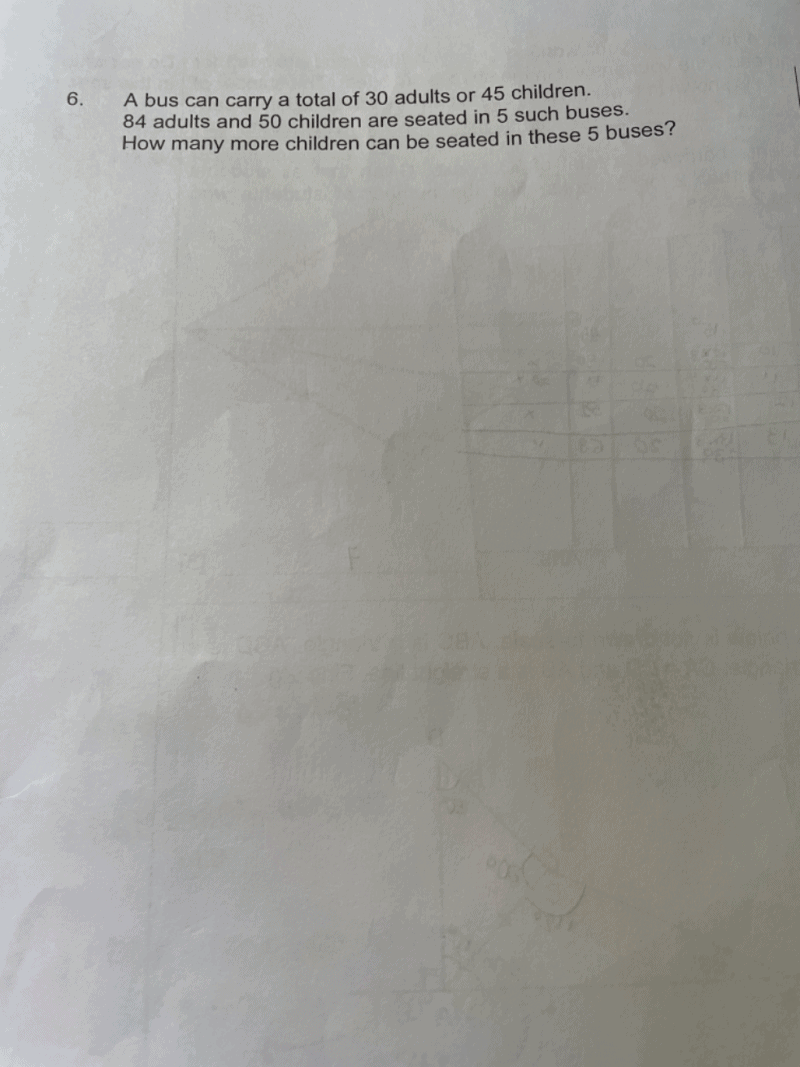# Questionis this assumption?

45 Children = 90 units

1 Child = 2 units

1 Bus = 90 units

5 Buses = 450 units

84 Adults + 50 Children = 352 units

450 units – 352 units = 48 units

48 units divide by 2 units = 24 more children

450 units – 352 units = 98 units

Number of additional children = 98 units / 2 units = 49

Alternatively, use ratio concept:

First we need to have an understanding that in this instance, the capacity is likely based on the load ie mass of individual and not number of seats available.

We could find the total number of children that all the buses could take:

5 x 45 children = 225 children.

Since we are given 84 adults and 50 children are already seated, we need to find the equivalent number of children for the seated adults.

To do this we use the given ratio of adults : children for each bus = 30 : 45 = 2 : 3

This means that for every 2 adults, an equivalent number of 3 children can be seated (ie the mass of 2 adults = mass of 3 children)

2 : 3 = 84 : 126 ie the 84 adults seated is equivalent to 126 children seated.

So the equivalent total number of children seated = 50  + 126 = 176

Therefore,  number of additional children to be seated = 225 – 176 = 49

0 Replies 0 Likes